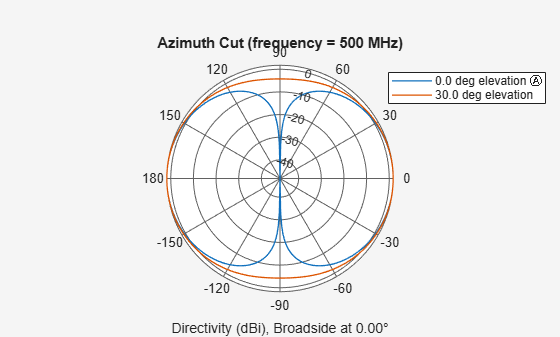Documentation

### This is machine translation

Mouseover text to see original. Click the button below to return to the English version of the page.

# patternAzimuth

System object: phased.ShortDipoleAntennaElement
Package: phased

Plot short-dipole antenna element directivity or pattern versus azimuth

## Syntax

```patternAzimuth(sElem,FREQ) patternAzimuth(sElem,FREQ,EL) patternAzimuth(sElem,FREQ,EL,Name,Value) PAT = patternAzimuth(___) ```

## Description

`patternAzimuth(sElem,FREQ)` plots the 2-D element directivity pattern versus azimuth (in dBi) for the element `sElem` at zero degrees elevation angle. The argument `FREQ` specifies the operating frequency.

`patternAzimuth(sElem,FREQ,EL)`, in addition, plots the 2-D element directivity pattern versus azimuth (in dBi) at the elevation angle specified by `EL`. When `EL` is a vector, multiple overlaid plots are created.

`patternAzimuth(sElem,FREQ,EL,Name,Value)` plots the element pattern with additional options specified by one or more `Name,Value` pair arguments.

`PAT = patternAzimuth(___)` returns the element pattern. `PAT` is a matrix whose entries represent the pattern at corresponding sampling points specified by the `'Azimuth'` parameter and the `EL` input argument.

## Input Arguments

expand all

Short-dipole antenna element, specified as a `phased.ShortDipoleAntennaElement` System object.

Example: `sElem = phased.ShortDipoleAntennaElement;`

Frequency for computing directivity and pattern, specified as a positive scalar. Frequency units are in hertz.

• For an antenna or microphone element, `FREQ` must lie within the range of values specified by the `FrequencyRange` or the `FrequencyVector` property of the element. Otherwise, the element produces no response and the directivity is returned as `–Inf`. Most elements use the `FrequencyRange` property except for `phased.CustomAntennaElement` and `phased.CustomMicrophoneElement`, which use the `FrequencyVector` property.

• For an array of elements, `FREQ` must lie within the frequency range of the elements that make up the array. Otherwise, the array produces no response and the directivity is returned as `–Inf`.

Example: `1e8`

Data Types: `double`

Elevation angles for computing sensor or array directivities and patterns, specified as a 1-by-N real-valued row vector. The quantity N is the number of requested elevation directions. Angle units are in degrees. The elevation angle must lie between –90° and 90°.

The elevation angle is the angle between the direction vector and the xy plane. When measured toward the z-axis, this angle is positive.

Example: `[0,10,20]`

Data Types: `double`

### Name-Value Pair Arguments

Specify optional comma-separated pairs of `Name,Value` arguments. `Name` is the argument name and `Value` is the corresponding value. `Name` must appear inside quotes. You can specify several name and value pair arguments in any order as `Name1,Value1,...,NameN,ValueN`.

Displayed pattern type, specified as the comma-separated pair consisting of `'Type'` and one of

• `'directivity'` — directivity pattern measured in dBi.

• `'efield'` — field pattern of the sensor or array. For acoustic sensors, the displayed pattern is for the scalar sound field.

• `'power'` — power pattern of the sensor or array defined as the square of the field pattern.

• `'powerdb'` — power pattern converted to dB.

Example: `'powerdb'`

Data Types: `char`

Azimuth angles, specified as the comma-separated pair consisting of `'Azimuth'` and a 1-by-P real-valued row vector. Azimuth angles define where the array pattern is calculated.

Example: `'Azimuth',[-90:2:90]`

Data Types: `double`

## Output Arguments

expand all

Element directivity or pattern, returned as an P-by-N real-valued matrix. The dimension P is the number of azimuth values determined by the `'Azimuth'` name-value pair argument. The dimension N is the number of elevation angles, as determined by the `EL` input argument.

## Examples

expand all

Specify a short-dipole antenna element having a direction along the y-axis. Then, plot an azimuth cut of the directivity at 0 and 30 degrees elevation. Assume the operating frequency is 500 MHz.

Create the antenna element.

```fc = 500e6; sSD = phased.ShortDipoleAntennaElement('FrequencyRange',[100,900]*1e6,... 'AxisDirection','y'); patternAzimuth(sSD,fc,[0 30])```Plot a reduced range of azimuth angles using the `Azimuth` parameter. Notice the change in scale.

`patternAzimuth(sSD,fc,[0 30],'Azimuth',[-20:20])`